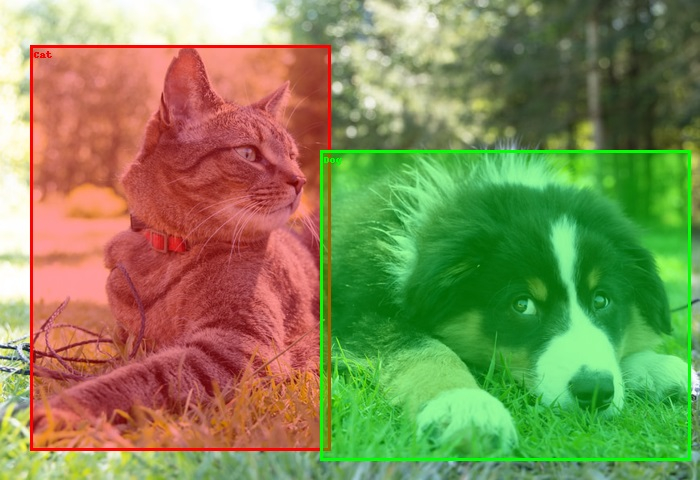# How to draw bounding boxes on an image in PyTorch?

The torchvision.utils package provides the draw_bounding_boxes() function to draw bounding boxes on an image. It supports images of type torch Tensor with shape (C x H x W) where C is the number of channels, and W and H are the width and height of the image, respectively.

If we read an image using Pillow or OpenCV, then we would have to first convert it to a torch tensor. We can draw one or more bounding boxes on the image. This function returns an image Tensor of dtype uint8 with bounding boxes drawn.

The bounding boxes should be torch Tensors of size [N,4], where N is the number of bounding boxes to be drawn. Each bounding box should contain four points in (xmin, ymin, xmax, ymax) format. In other words: 0 ≤ xmin < xmax < W, and 0 ≤ ymin < ymax < H.

We can also put labels on the bounding boxes. We can adjust the color and width of the bounding boxes. Also, we can fill the bounding box area with a specified color.

## Syntax

torch.utils.draw_bounding_boxes(image, boxes)

## Parameters

• image - image of type Tensor of shape (C x H x W).

• boxes - Tensor of size [N,4] containing bounding boxes coordinates in (xmin, ymin, xmax, ymax) format.

It also accepts more optional parameters such as labels, colors, fill, width, etc.

## Output

It returns an Image Tensor of size [C,H,W] with bounding boxes drawn.

## Steps

Import the required libraries. In all the following examples, the required Python libraries are torch and torchvision. Make sure you have already installed them.

import torch
import torchvision
from torchvision.utils import draw_bounding_boxes
• Read a JPEG or PNG image using image_read() function. Specify the full image path with image types (.jpg or .png). The output of this function is a torch tensor of size [image_channels, image_height, image_width].

img = read_image('cat.png')
• Define the bounding box as a torch tensor. The bounding box tensor should be of dtype torch.int. Unsqueeze the tensor if only one bounding box has to be drawn.

bbox = [290, 115, 405, 385]
bbox = torch.tensor(bbox, dtype=torch.int)
• Draw a bounding box on the image using the draw_bounding_boxes() function. Optionally, assign the image with the bounding box drawn to a new variable.

img=draw_bounding_boxes(img, bbox, width=3, colors=(255,255,0))
• Convert the image tensor with the bounding box drawn to a PIL image and display it.

img = torchvision.transforms.ToPILImage()(img)
img.show()

## Input Images

We will use these images as the input files in the following examples.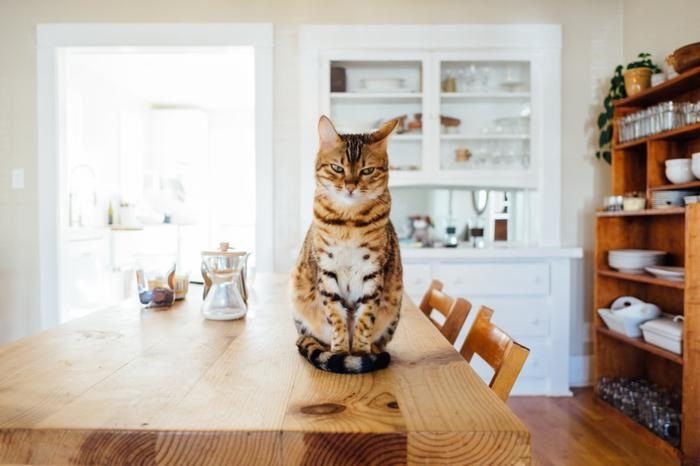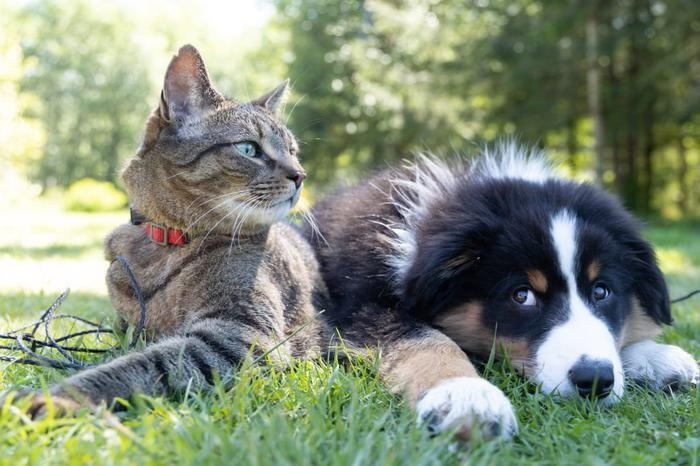## Example 1

The following program shows how to draw a bounding box on an image.

# Import the required libraries
import torch
import torchvision
from torchvision.utils import draw_bounding_boxes

# bounding box in (xmin, ymin, xmax, ymax) format
# top-left point=(xmin, ymin), bottom-right point = (xmax, ymax)
bbox = [290, 115, 405, 385]
bbox = torch.tensor(bbox, dtype=torch.int)
print(bbox)
print(bbox.size())
bbox = bbox.unsqueeze(0)
print(bbox.size())

# draw bounding box on the input image
img=draw_bounding_boxes(img, bbox, width=3, colors=(255,255,0))

# transform it to PIL image and display
img = torchvision.transforms.ToPILImage()(img)
img.show()

## Output

tensor([290, 115, 405, 385], dtype=torch.int32)
torch.Size()
torch.Size([1, 4])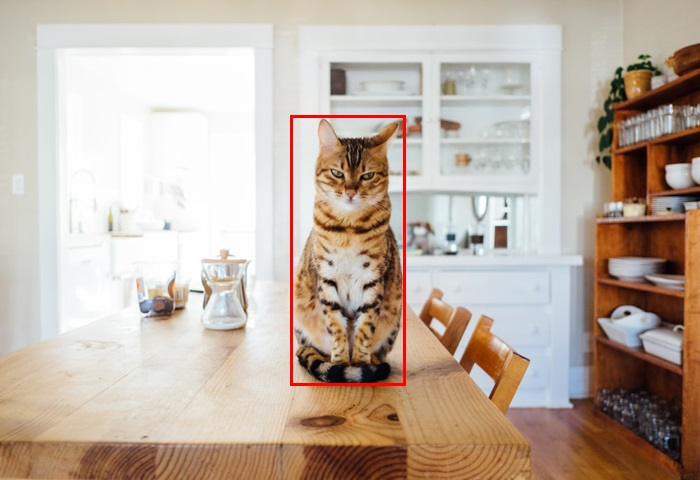## Example 2

The following program shows how to draw multiple bounding boxes on an image.

import torch
import torchvision
from torchvision.utils import draw_bounding_boxes

# bounding box in (xmin, ymin, xmax, ymax) format
bbox1 = [30, 45, 330, 450]
bbox2 = [320, 150, 690, 460]
bbox = [bbox1, bbox2]
bbox = torch.tensor(bbox, dtype=torch.int)
print(bbox)
print(bbox.size())

# draw bounding boxes on the input image
img=draw_bounding_boxes(img, bbox, width=3,
colors=[(255,0,0),(0,255,0)])
img = torchvision.transforms.ToPILImage()(img)
img.show()

## Output

tensor([[ 30, 45, 330, 450],
[320, 150, 690, 460]], dtype=torch.int32)
torch.Size([2, 4])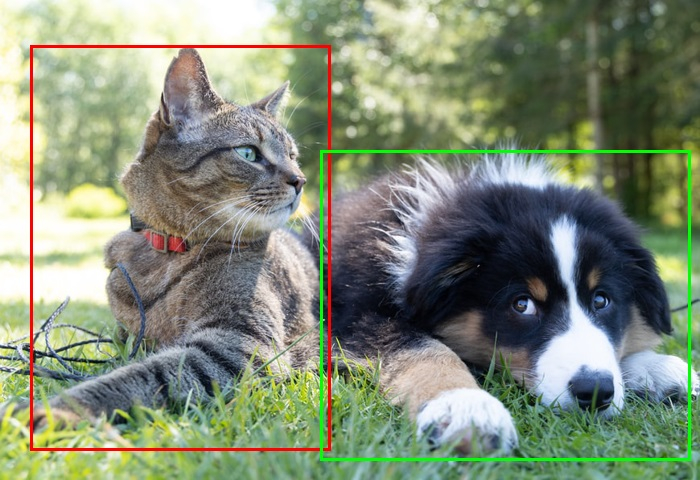## Example 3

The following program shows how to draw and fill multiple bounding boxes on an image.

import torch
import torchvision
from torchvision.utils import draw_bounding_boxes

# bounding box in (xmin, ymin, xmax, ymax) format
bbox1 = [30, 45, 330, 450]
bbox2 = [320, 150, 690, 460]
bbox = [bbox1, bbox2]
labels = ['Cat', 'Dog']
bbox = torch.tensor(bbox, dtype=torch.int)
print(bbox)
print(bbox.size())

# draw bounding boxes with fill color
img=draw_bounding_boxes(img, bbox,width=3,labels= labels,colors=[(255,0,0),(0,255,0)],fill =True,font_size=20)
img = torchvision.transforms.ToPILImage()(img)
img.show()

## Output

tensor([[ 30, 45, 330, 450],
[320, 150, 690, 460]], dtype=torch.int32)
torch.Size([2, 4])